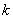## Quantitative Distance

Suppose we need to design some automation for a logistic company, which send some package from one city to another. Let us use four quantitative variables to distinguish one object from another. The four features are cost (rounded to smallest thousand of dollar), time (in hour), weight (in kg) and incentive (rounded to hundred of dollar, negative means punishment due to delay).

 Featurescost time weight incentive Object A 0 3 4 5 Object B 7 6 3 -1

We can represent the two objects as points in 4 dimension. Point A has coordinate (0, 3, 4, 5) and point B has coordinate (7, 6, 3, -1). Dissimilarity (or similarity) between the two objects are based on these coordinates.

Euclidean Distance
City block (Manhattan) distance
Chebyshev Distance
Minkowski Distance
Canberra distance
Bray Curtis distance (Sorensen distance)
Angular separation
Correlation coefficient

Preferable reference for this tutorial is

Teknomo, Kardi (2015) Similarity Measurement. http:\people.revoledu.comkardi tutorialSimilarity